# Quiz 9: Depreciation and Corporate Taxes

Economic depreciation can be defined as the reduction in the price of the stock of a firm, company or country either due to physical wear and tear, changes in the buying pattern or unfashionableness. The book value is the amount or the value of an asset as mentioned in the firm accounts. It is given that the cost price of the machine 4 years ago was \$30,000. The book value is \$9,369 and it can be sold for \$12,000. The life remaining of the asset is 3 years. The present value of economic depreciation can be computed as shown below:Hence, the present value of economic depreciation is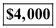.
Simple line depreciation is considered as the most simple and best method of depreciation. To calculate the annual depreciation charge, a simple formula is used which is shown below: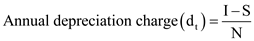Where I represent cost of the equipment, S represents salvage value and N represents the life of the project. The question requires us to calculate the depreciation of a new machine on every 31 st December for the years 2010, 2013 and 2016 where the cost of the machine is \$82,000 and its installation cost is \$3,000. Machine has expected useful life of 10 years, its salvage value is \$3,000. Book depreciation expense for 2010 can be calculated as given below:Hence, the Book depreciation expense for 2010 is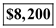. Calculation for the book value of the machine for the next 3 years is given in the table mentioned below: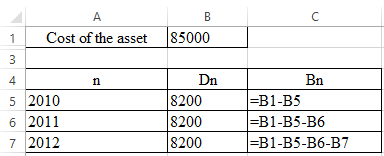Calculated book values are found to be: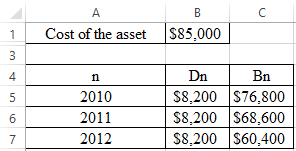Now, it is required to calculate the depreciation expenses for 2013. In 2013, \$8,000 was spent on repairing of machine. The calculations for depreciation are given below:Hence, the Book depreciation expense for 2013 is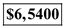. Calculation for the book value of the machine for the next 3 years is given in the table mentioned below: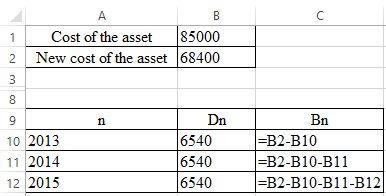Calculated book values are found to be: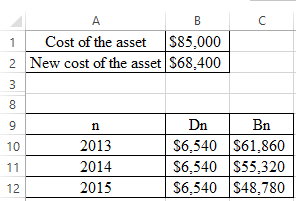Now, it is required to calculate the depreciation expenses for 2016. On 2 nd January 2016, \$5,000 was spent in order to make improvements in machine which lead to increase in its salvage value to \$6,000. So, the depreciation expense would be:Hence, the Book depreciation expense for 2016 is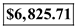.